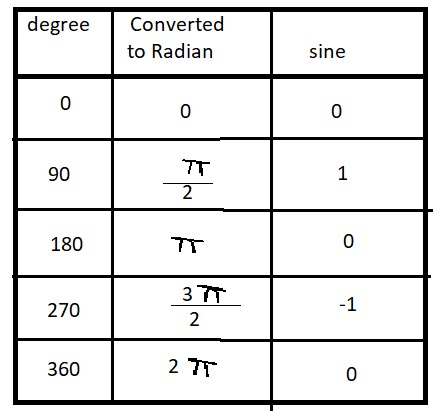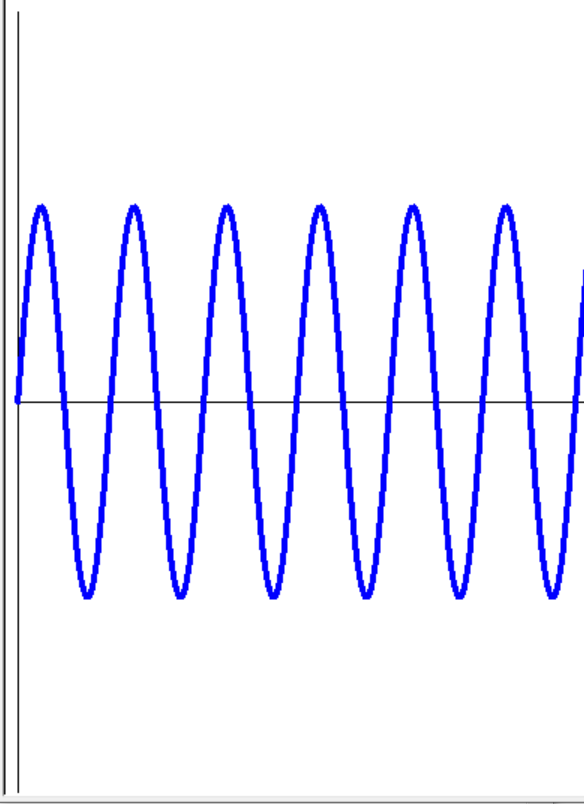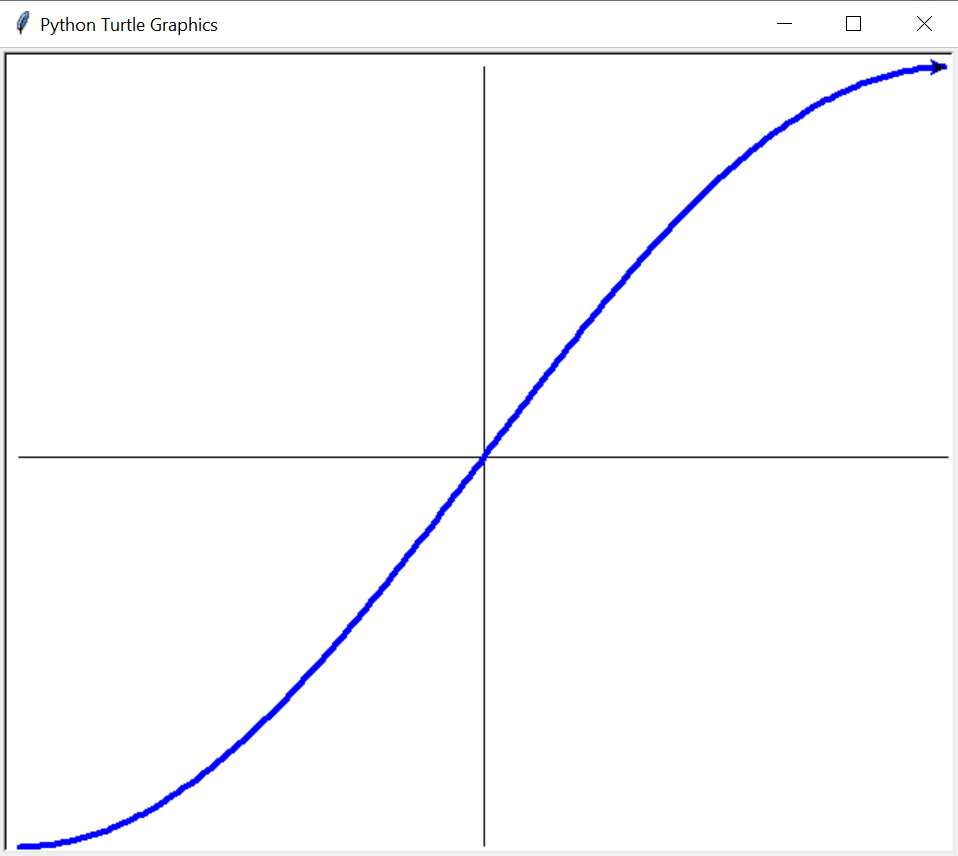Open In App

# Draw a Sine wave using Turtle in Python

In this article, we will draw a sinewave using a turtle in Python.

Turtle is one of the modules in python, it is a graphic that refers to controlling a graphical entity in a graphics window with x, and y coordinates. It is a toolkit that provides a simple and enjoyable way to draw pictures and shapes on the windows screen. By using turtles we can design any form, graphics. If you want to write code using turtle, you need to import the turtle.m

## What is a sine wave?

It is a pattern generated in a medium, when a disturbance (energy) travels from one point to another point, with the transport of particles known as a wave. A waveform described by the sine function, possibly shifted by some phase is known as a sine wave. It is the purest form of tone, which represents an exact frequency or a total value no natural source of sound generates a singular sine wave, but rather a magnitude of sine waves. It can truly be thought of as the building block of audio, like a piece of lego.  and it is used in many ways like radio waves, tides, musical tones, and electrical currents.
Here, the sine function is equal to the opposite side divided by the hypotenuse

`sin θ =  (Opposite side to θ / Hypotenuse)`

The sinewave graph and degree value are shown below:## Python3

 `import` `math``import` `turtle` `win ``=` `turtle.Screen()``win.bgcolor(``"white"``)` `# coordinate setting``win.setworldcoordinates(``0``, ``-``2``, ``3600``, ``2``)``t ``=` `turtle.Turtle()` `# Draw a vertical line``t.goto(``0``, ``2``)``t.goto(``0``, ``-``2``)``t.goto(``0``, ``0``)` `# Draw a Horizontal line``t.goto(``3600``, ``0``)``t.penup()``t.goto(``0``, ``0``)``t.pendown()``t.pencolor(``"blue"``)``t.pensize(``4``)` `# Generate wave form``for` `x ``in` `range``(``3600``):``    ``y ``=` `math.sin(math.radians(x))``    ``t.goto(x, y)`

Output:## What inverse sine Function

It is reciprocal of sine wave .sine inverse of the same ratio will give the measure of the angle.  y= sin -1(x) <=> sin y = x. Here, the sine function is equal to the opposite side divided by the hypotenuse, and Each range value (-1 to 1) is within the limited domain (-π/2, π/2)

`θ = Sin-1 (Opposite side to θ/Hypotenuse)`

The inverse sinewave graph and degree value table are shown below:

## Python3

 `import` `math``import` `turtle` `win ``=` `turtle.Screen()``win.bgcolor(``"white"``)` `# coordinate setting``win.setworldcoordinates(``-``90``, ``-``1``, ``90``, ``1``)``t ``=` `turtle.Turtle()` `# Draw a Horizontal line``t.goto(``-``90``, ``0``)``t.goto(``90``, ``0``)``t.penup()``t.goto(``0``, ``0``)``t.pendown()` `# Draw a vertical line``t.goto(``0``, ``1``)``t.goto(``0``, ``-``1``)``t.penup()``t.goto(``-``90``, ``-``1``)``t.pendown()``t.pencolor(``"blue"``)``t.pensize(``4``)` `# Generate wave form``for` `x ``in` `range``(``-``90``, ``90``):``    ``y ``=` `math.sin(math.radians(x))``    ``t.goto(x, y)`

Output: